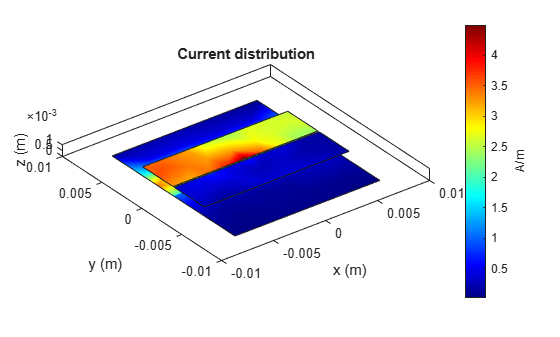coupledMicrostripLine

Create coupled microstrip transmission line

Description

Use the coupledMicrostripLine object to create a coupled microstrip transmission line. Coupled microstrip transmission lines are used to design directional couplers and filters. The combination of even and odd mode impedances determines the coupling ratio between the direct arm and the coupled arm.Creation

Description

example

coupledmicrostrip = coupledMicrostripLine creates a default coupled microstrip transmission line with a Teflon substrate. The default properties are for a resonating frequency of 1.5 GHz.

example

coupledmicrostrip = coupledMicrostripLine(Name=Value) sets Properties using one or more name-value arguments. For example, coupledMicrostripLine(Length=0.0300) creates a coupled microstrip transmission line of length 0.0300 meters.

Properties

expand all

Length of the coupled microstrip line in meters, specified as a positive scalar.

Example: coupledmicrostrip = coupledMicrostripLine(Length=0.0300)

Data Types: double

Width of the coupled microstrip line in meters, specified as a positive scalar.

Example: coupledmicrostrip = coupledMicrostripLine(Width=0.0041)

Data Types: double

Distance between the direct arm and the coupled arm of the coupled microstrip transmission line, specified as a positive scalar in meters.

Example: coupledmicrostrip = coupledMicrostripLine(Spacing=0.00300)

Data Types: double

Height of the coupled microstrip line from the ground plane, specified as a positive scalar in meters. In the case of a multilayer substrate, use the Height property to create a coupled microstrip line at the interface of the two dielectrics.

Example: coupledmicrostrip = coupledMicrostripLine(Height=0.0023)

Data Types: double

Width of the ground plane in meters, specified as a positive scalar.

Example: coupledmicrostrip = coupledMicrostripLine(GroundPlaneWidth=0.0400)

Data Types: double

Type of the dielectric material used as a substrate, specified as a dielectric object. The thickness of the default dielectric material Teflon is 0.0016 m or the same as the Height property.

Example: d = dielectric("FR4"); coupledmicrostrip = coupledMicrostripLine(Substrate=d)

Data Types: string | char

Type of metal used in the conducting layers, specified as a metal object.

Example: m = metal("PEC"); coupledmicrostrip =coupledMicrostripLine(Conductor=m)

Data Types: string | char

Object Functions

 charge Calculate and plot charge distribution current Calculate and plot current distribution design Design coupled microstrip transmission line around particular frequency feedCurrent Calculate current at feed port getZ0 Calculate characteristic impedance of transmission line layout Plot all metal layers and board shape mesh Change and view mesh properties of metal or dielectric in PCB component shapes Extract all metal layer shapes of PCB component show Display PCB component structure or PCB shape sparameters Calculate S-parameters for RF PCB objects

Examples

collapse all

Create and view a default coupled microstrip line.

coupledmicrostripline = coupledMicrostripLine
coupledmicrostripline =
coupledMicrostripLine with properties:

Length: 0.0271
Width: 0.0051
Spacing: 0.0046
Height: 0.0016
GroundPlaneWidth: 0.0300
Substrate: [1x1 dielectric]
Conductor: [1x1 metal]

show(coupledmicrostripline)Design and view a coupled microstrip line at the interface of a multilayer dielectric.

coupledmicrostripline = design(coupledMicrostripLine,4e9,Z0e=75,Z0o=36);
coupledmicrostripline.Substrate = dielectric(Name=["Teflon","Teflon"],EpsilonR=[2.1 2.1], ...
LossTangent=[0 0],Thickness=[0.8e-3 0.8e-3]);
coupledmicrostripline.Height = 0.8e-3;
show(coupledmicrostripline)Plot the current and charge distribution on the transmission line.

current(coupledmicrostripline,4e9)figure
charge(coupledmicrostripline,4e9)expand all

 Pozar, David M. Microwave Engineering. 4th ed. Hoboken, NJ: Wiley, 2012.

 “Microwaves101 | Coupled Line Couplers.” Accessed July 7, 2021. https://www.microwaves101.com/encyclopedias/coupled-line-couplers.

RF PCB Toolbox DocumentationGet trial now